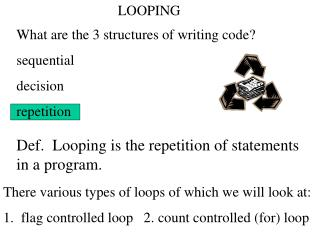DownloadDownload PresentationLOOPING

# LOOPING

Télécharger la présentation## LOOPING

- - - - - - - - - - - - - - - - - - - - - - - - - - - E N D - - - - - - - - - - - - - - - - - - - - - - - - - - -
##### Presentation Transcript

1. LOOPING What are the 3 structures of writing code? sequential decision repetition Def. Looping is the repetition of statements in a program. There various types of loops of which we will look at: 1. flag controlled loop 2. count controlled (for) loop

2. end start flag = false false true body of loop !flag == ? update flag Loop controlled by a boolean flag* and a pretest The flow of logic of a loop is shown in a flowchart: *sometimes called a sentinel

3. boolean done = false; //initialization step while ( ! done ) //loop condition { statements //body of loop . . . If (something) done = true; } //update flag based //on some decision //sometimes from user NOTE: this is a pretest loop the body is executed only when the loop condition is TRUE.

4. Example of a while loop using a flag: {char ch; boolean done = false; int num = 0; while (! done) { System.out.println(“Enter a number or -1 to quit:”); num = Input.getInt(); if (num = = -1) done = true; }

5. for ( lcv = initial value; lcv < final value; lcv ++ ) { /*statements in body of loop go here*/ } Count Controlled loops using the ‘For’ structure Interpretation: initialize ct; while ct < final value; execute body of loop; increment ct.

6. Summation is a common use of looping. Let’s write a loop to find the sum of the integer 1 to 10. int sum = 0; // called the accumulator int count = 0; // initialization of loop control variable while ( count < 10 ) // loop condition {sum = sum + count; // or sum += count count ++; // or count = count + 1 } //end while

7. Rewrite the above while loop as a For loop. What does this loop do? for (int x = 0; x < 32,768; x++); nothing but count to 32,768 - this provides a pause. (It is also a common error: do not put a semi-colon after the parentheses unless you want a loop that does nothing.)

8. Caution: infinite loop What does this do? For (ct = 10; ct != 20; ct +=3 ) { println ( “Hi!”); } Trace: ct output 10 Hi 13 Hi 16 Hi 19 Hi 22 Hi

9. Is a for loop a pretest loop, posttest loop or neither? Pretest. Write a loop whose body will never be executed. Can the lcv be any type besides int? yes. Any ordinal type: any primitve data type whose elements can be listed in sequential order. (short, int, long, char)

10. What does this loop do: for ( char ch = ‘A’; ch <= ‘Z’; ch++) displayResult(ch + “ “ + (int) ch); A 65 B 66 … Z 90